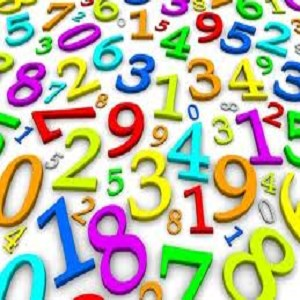Free
Overview System Requirements Related

Description

A number is a mathematical object used to count and measure. In mathematics, the definition of number has been extended over the years to include such numbers as zero, negative numbers, rational numbers, irrational numbers, and complex numbers.Mathematical operations are certain procedures that take one or more numbers as input and produce a number as output. Unary operations take a single input number and produce a single output number. For example, the successor operation adds one to an integer, thus the successor of 4 is 5. Binary operations take two input numbers and produce a single output number. Examples of binary operations include addition, subtraction, multiplication, division, and exponentiation. The study of numerical operations is called arithmetic.A notational symbol that represents a number is called a numeral. In addition to their use in counting and measuring, numerals are often used for labels (telephone numbers), for ordering (serial numbers), and for codes (e.g., ISBNs).In common use, the word number can mean the abstract object, the symbol, or the word for the number.

People also like

Dinesh V T

Dinesh V T

24/08/2012

1.3 MB

Age rating

For ages 3 and up

Installation

Get this app while signed in to your Microsoft account and install on up to ten Windows 10 devices.

Language supported

English (United States)